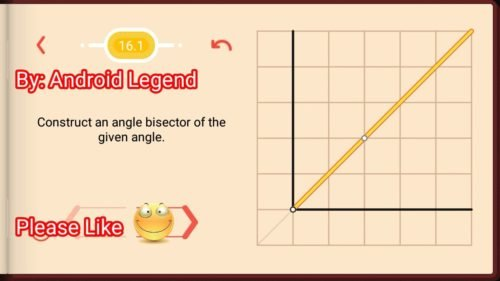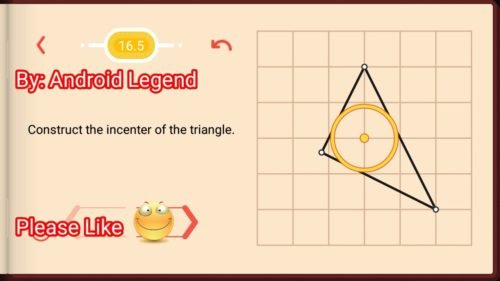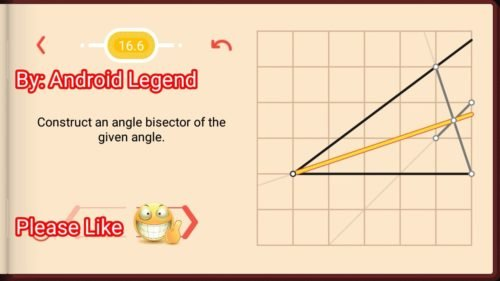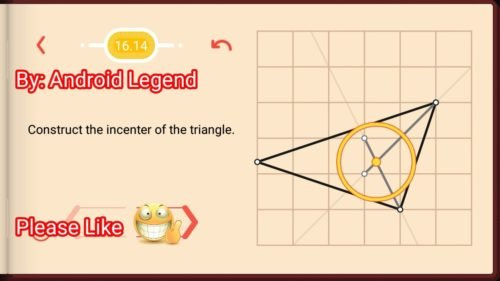# Pythagorea Level 16.1 16.2 16.3 16.4 16.5 16.6 16.7 16.8 16.9 16.10 16.11 16.12 16.13 16.14 16.15 16.16 Solution/AnswersPythagorea is android/iOS app developed by Horis International Limited. Solutions hints and answers to pythagorea are available in this post scroll down to find solutions to all the levels.

This game is mostly focused on geometric puzzles and construction. the workspace is divided into grids to draw lines. You should know all the basic Math operations. All lines and shapes are drawn on a grid whose cells are squares. Most of the game levels can be answered using natural intuition and bye some basic laws of geometry.

You have to connect points on the grid using straight lines to construct an element, you can even use intersection points to draw. some levels are very easy some are of medium difficulty and some are very hard to solve, that’s why I am providing solutions to all the problems.

If you have forgotten basic course you did in your elementary education, this game is for you to revise all the concept using a single game or if you don’t know any of the geometry this game features “i” button from where you can learn about all the shapes and geometry and then play this game to enhance your geometry skill.And do not miss your chance to familiarize children with mathematics. Pythagorea is an excellent way to make friends with geometry and benefit from spending time together.

If you are here for levels other than ‘Angle Bisectors’ Go to directory of all other levels at : https://www.puzzlegamemaster.com/pythagorea-all-answers-solutions/

• Pythagorea Level 16.1: Construct an angle bisector of the given angle.• Pythagorea Level 16.2: Construct an angle bisector of the given angle.• Pythagorea Level 16.3: Construct the incenter of the triangle.• Pythagorea Level 16.4: Construct an angle bisector of the given angle.• Pythagorea Level 16.5: Construct the incenter of the triangle.• Pythagorea Level 16.6: Construct an angle bisector of the given angle.• Pythagorea Level 16.7: Construct an angle bisector of the given angle.• Pythagorea Level 16.8: Construct a bisector of the angle Whose vertex lies outside the field.• Pythagorea Level 16.9: Construct a bisector of the angle Whose vertex lies outside the field.• Pythagorea Level 16.10: Construct an angle bisector of the given angle.• Pythagorea Level 16.11: Construct the incenter of the triangle.• Pythagorea Level 16.12: Construct an angle bisector of the given angle.• Pythagorea Level 16.13: Construct a bisector of the angle Whose vertex lies outside the field.• Pythagorea Level 16.14: Construct the incenter of the triangle.• Pythagorea Level 16.15: Construct an angle bisector of the given angle.• Pythagorea Level 16.16: Construct an angle bisector of the given angle.Feel free to comment below if you have any doubts regarding the solutions. I will try to help you guys. All other levels are posted on this blog please visit them too.

### 6 thoughts on “Pythagorea Angle Bisectors All Levels (16.1-16.16) Solutions/Answers”

•September 8, 2018 at 2:55 pm

it is not maths, it is luck with no explaination at all.
maths is not finding a number but understanding a process.

•June 1, 2018 at 3:22 pm

Can you explain the solution for Pythagorea Level 16.16? Why does it work that way?

•January 28, 2019 at 9:38 pm

I struggled with 16.16 for a while and finally gave up. I’m trying to understand the logic behind the solution. I see that the line constructed in the solution, from the center of the circle, is parallel to the given ray, and therefore cuts the arc between the ray and the diameter line, perfectly in half, and therefore, the point where this line intersects the arc, gives you the bisector. But I can’t figure out how you know that to construct that parallel line, you intersect your radius line with the point defined by the intersection of that lower right hand “slice” line with the grid line.

•February 14, 2019 at 2:25 am

Don’t even need to construct anything in the “lower” part of the circle.

Lets call the centre O at (0,0)
Lets call the angle-point A at (sqrt(5),0)
Lets call the “upper” given intersection B at (-1,2)
Lets call the other given intersection C at (-sqrt(5),0)
FYI: the angle BAC is exactly half the angle BOC
Create point D halfway between B and C.
OD cuts the angle COB in half.
Lets call the new intersection between the circle and OD the point E.
(This means the angle BOD=BOE equals BAC)
That means OD cuts the segment CB in half at the point E.
The angle BOE is twice the angle BAE
Q.E.D.

•April 3, 2020 at 5:28 pm
•